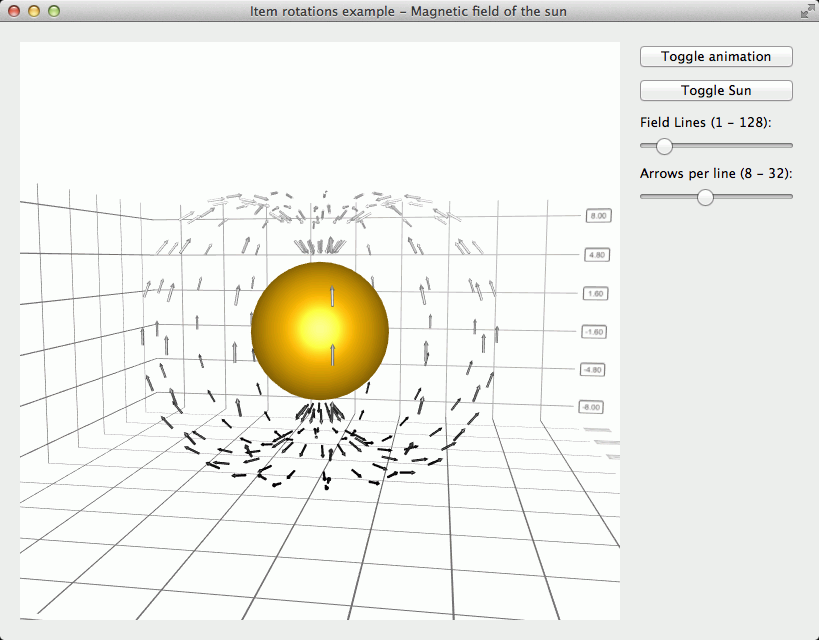# Rotations Example

Using rotated scatter items.

This example shows how to do the following:

• Use item rotations
• Use custom item meshes
• Use range gradient to color the series

For more basic example about using Qt Data Visualization graphs, see Bars Example.#### Running the Example

To run the example from Qt Creator, open the Welcome mode and select the example from Examples. For more information, visit Building and Running an Example.

#### Using Rotations

In this example we want to orient the arrow items tangentially to the origin. This requires rotating them, which can be achieved by specifying rotation quaternion to each item:

```QQuaternion yRotation = QQuaternion::fromAxisAndAngle(0.0f, 1.0f, 0.0f, horizontalAngle * radiansToDegrees);
QQuaternion zRotation = QQuaternion::fromAxisAndAngle(0.0f, 0.0f, 1.0f, verticalAngle * radiansToDegrees);
QQuaternion totalRotation = yRotation * zRotation;
ptrToDataArray->setRotation(totalRotation);```

Since the items need to be rotated along two axes, we define two rotation quaternions, one for Y-axis and one for Z-axis, and then multiply these together to get the total rotation, which we set to the data item.

#### Using Custom Item Meshes

The narrow arrow mesh we use for magnetic field arrow items is not a standard mesh. Instead we supply our own `narrowarrow.obj` file which contains the object definition for the mesh in `Wavefront` obj format:

```m_magneticField->setMesh(QAbstract3DSeries::MeshUserDefined);
m_magneticField->setUserDefinedMesh(QStringLiteral(":/mesh/narrowarrow.obj"));```

Setting the color style to range gradient in a series means that the item is colored according to its relative Y-value on the visible Y-coordinate range. We want the arrows on the bottom part of the graph to be darker and gradually get lighter higher they are, so we define a range gradient with black color at the position 0.0 and white color at the position 1.0:

```QLinearGradient fieldGradient(0, 0, 16, 1024);